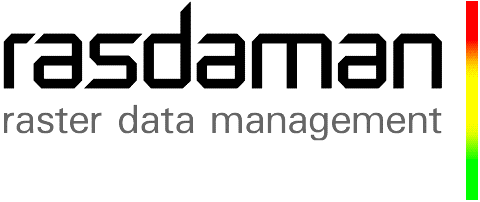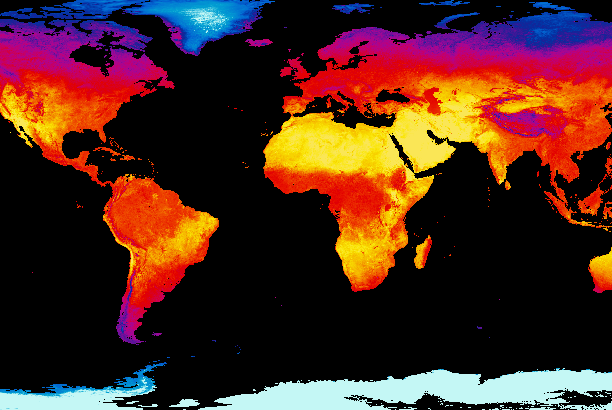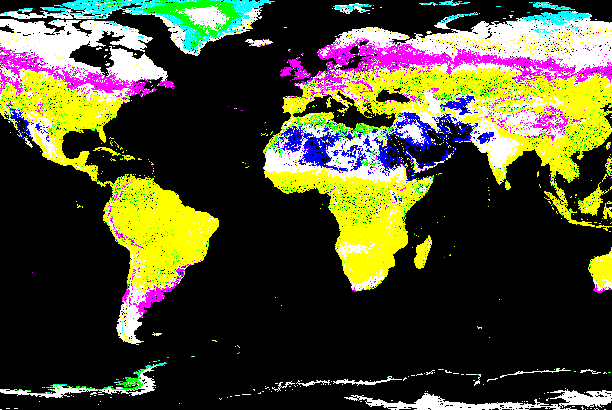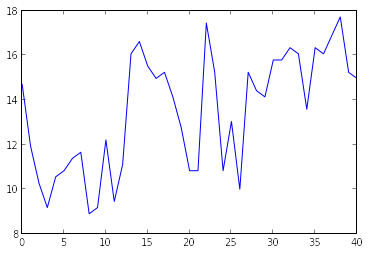This notebook gives a short introduction to the Open Geospatial Consortium (OGC) standard WCS (Web Coverage Service) and WCPS (Web Coverage Processing Service). It provides small examples help you to get started retrieving data from https://inspire.rasdaman.org/rasdaman/ows which hosts Rasdaman (Multidimensional Raster Database Manager) - http://rasdaman.org to process OGC standarized requests from data cubes.Data cube's terminology (multidimensional array)

### OGC WCS Requests¶

OGC Web Coverage Service (WCS, http://www.opengeospatial.org/standards/wcs) is a standarized data access protocol. There are 3 core requests (GetCapabilities, DescribeCoverage) in order to discover available data (coverages) on a server (and GetCoverage) to get them to your local system.

The WCS request can access to Rasdaman demo server via the url below - by specifying either one of the core requests or the WCS extension.
https://inspire.rasdaman.org/rasdaman/ows?service=WCS&version=2.0.1&request=...

• GetCapabilities

Returns XML-encoded description of service properties and all data (coverages) available on the server.

• The "Capabilities document" is returned and contains information e.g. service provider, to format encodings, supported interpolation methods, coverages on the server,...

• Describe Coverage

Returns XML-encoded description of a specific coverage, to check axes resolutions, dimensions etc.

• Returns the "Coverage description document" that contains information to spatial and temporal dimension and general information to the type of coverage.

• GetCoverage

Returns a full coverage or a subset by spatial / temporal dimensions of the coverage (slicing and trimming on coverage's axes)

• Returns a subset coverage from a 3D original coverage to 2D coverage by slicing on time axis and encode result in image/png.Trimming / Slicing on 3D data cube to get a subset coverage

### How to integrate WCS requests into a python script¶

You can install required Python libraries for demos with pip tool https://pypi.org/project/pip/.

In [ ]:
# Install required libraries
pip install requests
pip install owslib
pip install numpy

• Using requests library to send requests to server and parse result (if in XML) manually.
In [ ]:
# Using requests library to send GET request to server
import requests

result = requests.get("https://inspire.rasdaman.org/rasdaman/ows?service=WCS&version=2.0.1&request=GetCapabilities")

# Result is XML text (you need to parse it manually to extract valuable information
# such as: coverage Id, dimensions, axis labels,...)
print result.text

• Using OWSlib library as a more convenient way to parse data in XML for WCS requests
In :
# Get result of GetCapabilities request from Rasdaman demo server

from owslib.wcs import WebCoverageService

my_wcs = WebCoverageService("https://inspire.rasdaman.org/rasdaman/ows", version="2.0.1")

# Print all available coverages
print my_wcs.contents.keys()

['INSPIRE_EL', 'FiLCCoverageBit', 'INSPIRE_OI_RGB', 'INSPIRE_OI_IR', 'INSPIRE_WS_LC', 'AvgTemperatureColorScaled', 'AvgLandTemp']

In :
# Select a coverage (3D irregular coverage) to describe and set it to a reference variable
cov = my_wcs.contents["AvgTemperatureColorScaled"]

In :
# Get number of dimensions of coverage
print cov.grid.dimension

3

In :
# Get coverage axis labels with order according to coverage's Coordinate Reference System (CRS)
print cov.grid.axislabels

['Lat', 'Long', 'ansi']

In :
# Get coverage's time axis's domain (as this coverage is 3D with time axis)
print cov._getTimeLimits()

[datetime.datetime(2000, 2, 1, 0, 0), datetime.datetime(2015, 7, 1, 0, 0)]

In :
# Get coverage's grid lower limits
print cov.grid.lowlimits

['0', '0', '0']

In :
# Get coverage's grid upper limits
print cov.grid.highlimits

['449', '899', '184']

In :
# Get offset vectors of coverage (axes' resolutions are non-zero values, respectively)
print cov.grid.offsetvectors

[['-0.4', '0', '0'], ['0', '0.4', '0'], ['0', '0', '1']]

In :
# Get the list of coefficients for irregular axis (time is an irregular axis of this coverage)
print cov.timepositions

[datetime.datetime(2000, 2, 1, 0, 0), datetime.datetime(2000, 3, 1, 0, 0), datetime.datetime(2000, 4, 1, 0, 0), datetime.datetime(2000, 5, 1, 0, 0), datetime.datetime(2000, 6, 1, 0, 0), datetime.datetime(2000, 7, 1, 0, 0), datetime.datetime(2000, 8, 1, 0, 0), datetime.datetime(2000, 9, 1, 0, 0), datetime.datetime(2000, 10, 1, 0, 0), datetime.datetime(2000, 11, 1, 0, 0), datetime.datetime(2000, 12, 1, 0, 0), datetime.datetime(2001, 1, 1, 0, 0), datetime.datetime(2001, 2, 1, 0, 0), datetime.datetime(2001, 3, 1, 0, 0), datetime.datetime(2001, 4, 1, 0, 0), datetime.datetime(2001, 5, 1, 0, 0), datetime.datetime(2001, 6, 1, 0, 0), datetime.datetime(2001, 7, 1, 0, 0), datetime.datetime(2001, 8, 1, 0, 0), datetime.datetime(2001, 9, 1, 0, 0), datetime.datetime(2001, 10, 1, 0, 0), datetime.datetime(2001, 11, 1, 0, 0), datetime.datetime(2001, 12, 1, 0, 0), datetime.datetime(2002, 1, 1, 0, 0), datetime.datetime(2002, 2, 1, 0, 0), datetime.datetime(2002, 3, 1, 0, 0), datetime.datetime(2002, 4, 1, 0, 0), datetime.datetime(2002, 5, 1, 0, 0), datetime.datetime(2002, 6, 1, 0, 0), datetime.datetime(2002, 7, 1, 0, 0), datetime.datetime(2002, 8, 1, 0, 0), datetime.datetime(2002, 9, 1, 0, 0), datetime.datetime(2002, 10, 1, 0, 0), datetime.datetime(2002, 11, 1, 0, 0), datetime.datetime(2002, 12, 1, 0, 0), datetime.datetime(2003, 1, 1, 0, 0), datetime.datetime(2003, 2, 1, 0, 0), datetime.datetime(2003, 3, 1, 0, 0), datetime.datetime(2003, 4, 1, 0, 0), datetime.datetime(2003, 5, 1, 0, 0), datetime.datetime(2003, 6, 1, 0, 0), datetime.datetime(2003, 7, 1, 0, 0), datetime.datetime(2003, 8, 1, 0, 0), datetime.datetime(2003, 9, 1, 0, 0), datetime.datetime(2003, 10, 1, 0, 0), datetime.datetime(2003, 11, 1, 0, 0), datetime.datetime(2003, 12, 1, 0, 0), datetime.datetime(2004, 1, 1, 0, 0), datetime.datetime(2004, 2, 1, 0, 0), datetime.datetime(2004, 3, 1, 0, 0), datetime.datetime(2004, 4, 1, 0, 0), datetime.datetime(2004, 5, 1, 0, 0), datetime.datetime(2004, 6, 1, 0, 0), datetime.datetime(2004, 7, 1, 0, 0), datetime.datetime(2004, 8, 1, 0, 0), datetime.datetime(2004, 9, 1, 0, 0), datetime.datetime(2004, 10, 1, 0, 0), datetime.datetime(2004, 11, 1, 0, 0), datetime.datetime(2004, 12, 1, 0, 0), datetime.datetime(2005, 1, 1, 0, 0), datetime.datetime(2005, 2, 1, 0, 0), datetime.datetime(2005, 3, 1, 0, 0), datetime.datetime(2005, 4, 1, 0, 0), datetime.datetime(2005, 5, 1, 0, 0), datetime.datetime(2005, 6, 1, 0, 0), datetime.datetime(2005, 7, 1, 0, 0), datetime.datetime(2005, 8, 1, 0, 0), datetime.datetime(2005, 9, 1, 0, 0), datetime.datetime(2005, 10, 1, 0, 0), datetime.datetime(2005, 11, 1, 0, 0), datetime.datetime(2005, 12, 1, 0, 0), datetime.datetime(2006, 1, 1, 0, 0), datetime.datetime(2006, 2, 1, 0, 0), datetime.datetime(2006, 3, 1, 0, 0), datetime.datetime(2006, 4, 1, 0, 0), datetime.datetime(2006, 5, 1, 0, 0), datetime.datetime(2006, 6, 1, 0, 0), datetime.datetime(2006, 7, 1, 0, 0), datetime.datetime(2006, 8, 1, 0, 0), datetime.datetime(2006, 9, 1, 0, 0), datetime.datetime(2006, 10, 1, 0, 0), datetime.datetime(2006, 11, 1, 0, 0), datetime.datetime(2006, 12, 1, 0, 0), datetime.datetime(2007, 1, 1, 0, 0), datetime.datetime(2007, 2, 1, 0, 0), datetime.datetime(2007, 3, 1, 0, 0), datetime.datetime(2007, 4, 1, 0, 0), datetime.datetime(2007, 5, 1, 0, 0), datetime.datetime(2007, 6, 1, 0, 0), datetime.datetime(2007, 7, 1, 0, 0), datetime.datetime(2007, 8, 1, 0, 0), datetime.datetime(2007, 9, 1, 0, 0), datetime.datetime(2007, 10, 1, 0, 0), datetime.datetime(2007, 11, 1, 0, 0), datetime.datetime(2007, 12, 1, 0, 0), datetime.datetime(2008, 1, 1, 0, 0), datetime.datetime(2008, 2, 1, 0, 0), datetime.datetime(2008, 3, 1, 0, 0), datetime.datetime(2008, 4, 1, 0, 0), datetime.datetime(2008, 5, 1, 0, 0), datetime.datetime(2008, 6, 1, 0, 0), datetime.datetime(2008, 7, 1, 0, 0), datetime.datetime(2008, 8, 1, 0, 0), datetime.datetime(2008, 9, 1, 0, 0), datetime.datetime(2008, 10, 1, 0, 0), datetime.datetime(2008, 11, 1, 0, 0), datetime.datetime(2008, 12, 1, 0, 0), datetime.datetime(2009, 1, 1, 0, 0), datetime.datetime(2009, 2, 1, 0, 0), datetime.datetime(2009, 3, 1, 0, 0), datetime.datetime(2009, 4, 1, 0, 0), datetime.datetime(2009, 5, 1, 0, 0), datetime.datetime(2009, 6, 1, 0, 0), datetime.datetime(2009, 7, 1, 0, 0), datetime.datetime(2009, 8, 1, 0, 0), datetime.datetime(2009, 9, 1, 0, 0), datetime.datetime(2009, 10, 1, 0, 0), datetime.datetime(2009, 11, 1, 0, 0), datetime.datetime(2009, 12, 1, 0, 0), datetime.datetime(2010, 1, 1, 0, 0), datetime.datetime(2010, 2, 1, 0, 0), datetime.datetime(2010, 3, 1, 0, 0), datetime.datetime(2010, 4, 1, 0, 0), datetime.datetime(2010, 5, 1, 0, 0), datetime.datetime(2010, 6, 1, 0, 0), datetime.datetime(2010, 7, 1, 0, 0), datetime.datetime(2010, 8, 1, 0, 0), datetime.datetime(2010, 9, 1, 0, 0), datetime.datetime(2010, 10, 1, 0, 0), datetime.datetime(2010, 11, 1, 0, 0), datetime.datetime(2010, 12, 1, 0, 0), datetime.datetime(2011, 1, 1, 0, 0), datetime.datetime(2011, 2, 1, 0, 0), datetime.datetime(2011, 3, 1, 0, 0), datetime.datetime(2011, 4, 1, 0, 0), datetime.datetime(2011, 5, 1, 0, 0), datetime.datetime(2011, 6, 1, 0, 0), datetime.datetime(2011, 7, 1, 0, 0), datetime.datetime(2011, 8, 1, 0, 0), datetime.datetime(2011, 10, 1, 0, 0), datetime.datetime(2011, 11, 1, 0, 0), datetime.datetime(2011, 12, 1, 0, 0), datetime.datetime(2012, 1, 1, 0, 0), datetime.datetime(2012, 2, 1, 0, 0), datetime.datetime(2012, 3, 1, 0, 0), datetime.datetime(2012, 4, 1, 0, 0), datetime.datetime(2012, 5, 1, 0, 0), datetime.datetime(2012, 6, 1, 0, 0), datetime.datetime(2012, 7, 1, 0, 0), datetime.datetime(2012, 8, 1, 0, 0), datetime.datetime(2012, 9, 1, 0, 0), datetime.datetime(2012, 10, 1, 0, 0), datetime.datetime(2012, 11, 1, 0, 0), datetime.datetime(2012, 12, 1, 0, 0), datetime.datetime(2013, 1, 1, 0, 0), datetime.datetime(2013, 2, 1, 0, 0), datetime.datetime(2013, 3, 1, 0, 0), datetime.datetime(2013, 4, 1, 0, 0), datetime.datetime(2013, 5, 1, 0, 0), datetime.datetime(2013, 6, 1, 0, 0), datetime.datetime(2013, 7, 1, 0, 0), datetime.datetime(2013, 8, 1, 0, 0), datetime.datetime(2013, 9, 1, 0, 0), datetime.datetime(2013, 10, 1, 0, 0), datetime.datetime(2013, 11, 1, 0, 0), datetime.datetime(2013, 12, 1, 0, 0), datetime.datetime(2014, 1, 1, 0, 0), datetime.datetime(2014, 2, 1, 0, 0), datetime.datetime(2014, 3, 1, 0, 0), datetime.datetime(2014, 4, 1, 0, 0), datetime.datetime(2014, 5, 1, 0, 0), datetime.datetime(2014, 6, 1, 0, 0), datetime.datetime(2014, 7, 1, 0, 0), datetime.datetime(2014, 8, 1, 0, 0), datetime.datetime(2014, 9, 1, 0, 0), datetime.datetime(2014, 10, 1, 0, 0), datetime.datetime(2014, 11, 1, 0, 0), datetime.datetime(2014, 12, 1, 0, 0), datetime.datetime(2015, 1, 1, 0, 0), datetime.datetime(2015, 2, 1, 0, 0), datetime.datetime(2015, 3, 1, 0, 0), datetime.datetime(2015, 4, 1, 0, 0), datetime.datetime(2015, 5, 1, 0, 0), datetime.datetime(2015, 6, 1, 0, 0), datetime.datetime(2015, 7, 1, 0, 0)]

In :
import requests
from IPython.display import Image

# Get a subset coverage by slicing on time axis, trimming on Lat and Long axes, then encode result in image/png.
request = "&REQUEST=GetCoverage"
coverage_id = "&COVERAGEID=AvgTemperatureColorScaled"
subset_time = "&SUBSET=ansi(\"2002-09-01T00:00:00.000Z\")"
subset_lat = "&SUBSET=Lat(-81.7242,81.7825)"
subset_long = "&SUBSET=Lon(-122.1420,122.2185)"
encode_format = "&FORMAT=image/png"

response = requests.get("http://ows.rasdaman.org/rasdaman/ows?&SERVICE=WCS&VERSION=2.0.1"
+ request + coverage_id + subset_time + subset_lat + subset_long + encode_format)

# Display result directly (sea coastnear by Fiucimino)
Image(data=response.content)

Out:### OGC WCPS Requests¶

OGC Web Coverage Processing Service (WCPS, http://www.opengeospatial.org/standards/wcps) is an extension of OGC WCS standard which allows extraction, analysis and processing of multi-dimensional coverages. WCPS syntax tentatively has been crafted close to the XQuery language. It establishes a protocol to send a query string to a server and obtain, as a result of the server's processing, a set of coverages.

The so-called "processing expression" is applied on each of the coverages specified in the given list (coverageList), given that the optional boolean expression returns true when evaluated on the coverage. Each coverage is referred to in the query by the correspondent identifier variableName in the processing expression. A processing expression branches down to a miscellanea of possible sub-expressions that are able to return either scalars (scalar expressions) or encoded marrays (coverage expressions) and operate on both the data and metadata of a coverage. More details at http://tutorial.rasdaman.org/rasdaman-and-ogc-ws-tutorial/#ogc-web-services-web-coverage-processing-service.

Queries can be executed by using following request (ProcessCoverages is an extension of WCS request):

With query parameter is a WCPS query with valid syntax for server to process, example of subsetting a 3D coverage to 2D coverage and encode result in image/png as same as the previous demo for WCS GetCoverage request:

In [ ]:
# NOTE: for GET requests, WCPS query cannot contain new lines character (below query is just for brevity)
for $c in (AvgTemperatureColorScaled) return encode($c[Lat(-81.7242:81.7825),
Lon(-122.1420:122.2185),
ansi("2002-09-01T00:00:00.000Z")],
"png")


WCPS enables for much more complex processing requests than WCS can offer. For example, time-series processing to findout the difference between 2 date-time slices ("2002-09-01T00:00:00.000Z" (reference) and "2009-05-01T00:00:00.000Z") of coverage AvgTemperatureColorScaled.

NOTE: In case of using WCPS queries with special characters like '[', ']', '{', '}', it must use POST requests.

In :
import requests

query = '''
for $c in (AvgTemperatureColorScaled) return encode( ($c[Lat(-81.7242:81.7825), Lon(-122.1420:122.2185), ansi("2002-09-01T00:00:00.000Z")]
- $c[Lat(-81.7242:81.7825), Lon(-122.1420:122.2185), ansi("2009-05-01T00:00:00.000Z")]) * 200, "png") ''' # Send a WCPS with special characters to server in POST request response = requests.post('http://ows.rasdaman.org/rasdaman/ows', data = {'query': query}) # Display result directly Image(data=response.content)  Out:### Further multi-dimensional array processing With Python Libraries¶ Output data from rasdaman can be used for other Python libraries to process / visualize such as Numpy. Select the proper encode format which fits with your application in advanced scenarios. In : %matplotlib inline import requests import numpy as np import matplotlib.pyplot as pp # Select a 1D subset coverage result encoded in JSON query = '''for$c in (AvgLandTemp) return encode(
\$c[Lat(41.7242:45.7352),
Long(12.1420),
ansi("2000-02-01T00:00:00.000Z")]
, "json")
'''

response = requests.post('http://ows.rasdaman.org/rasdaman/ows', data = {'query': query})

# Convert 1D result in JSON to Numpy 1D array
array = np.array(eval(response.text))

# Display the raw values of 1D Numpy 1D array in a plot
pp.plot(array)
pp.show()